# T Flip Flops: What is it? (Truth Table, Circuit And Timing Diagram)

Contents

## What is a T Flip Flop?

A T flip flop is known as a toggle flip flop because of its toggling operation. It is a modified form of the JK flip flop. A T flip flop is constructed by connecting J and K inputs, creating a single input called T. Hence why a T flip flop is also known as a single input JK flip flop.

The defining characteristic of T flip flop is that it can change its output state. You can change the output signal from one state (on or off) to another state (off or on).

The clock signal must set high to toggle the output. When the clock is set low, the output remains as it is whether the input signal is set high or low. So, to change the output condition, the clock signal has to be high.

The graphical symbol of T flip flop is as shown in the below figure.

## T Flip Flop Truth Table

T flip flop is a single input flip flop. Along with this input, we need to give a clock signal to the flip flop. The T flip flop only works when a clock signal is high.

When the T signal is set low (0), it will not affect the present state of the output and the response will not change.

When the T signal is set high (1), if the present output status is also high (1), it converts into low (0); if the present output status is low (0), it converts it into high (1). It means it will toggle the output signal.

The below table shows the truth table of T flip flop. In this truth table, we have assumed that the clock signal is set high for all operations. Qn represents the present state and Qn+1 represents the next state of the output.

## T Flip Flop Circuit

There is no IC available for the T flip flop. Generally, it is modified from the JK flip flop. The most common IC used to make T flip flop is MC74HC73A (Dual JK Flip Flop).

T flip flop can be derived from JK, SR, and D flip flop. The easiest way to construct a T flip flop is from a JK flip flop.

### T Flip Flop Using JK Flip Flop

In this method, we need not any extra gates to make T flip flop. The input J and K are connected and make one input terminal. This input terminal is named T input.

We have a truth table of T flip flop as shown in table-1. And we know the excitation table of JK Flip flop as shown in below table-2.

Now, combine the characteristic table of T Flip flop and the excitation table of JK Flip flop.

From the above table, make a K-map for J and K inputs.

K-map for input J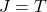K-map for input K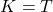From the above K-map and equations, if you give common input to J and K of JK flip flop, it will behave like T Flip flop. Hence, the circuit diagram of T flip flop from JK flip flop is as shown in the below figure.

### T Flip Flop Using SR Flip Flop

The circuit diagram of T flip flop can be derived from SR Flip flop. And for that, we will do the same process as above.

Therefore, we need the truth table of T flip flop (table-1) and excitation table of SR flip flop (as shown in below table-3).

Now, we combine both tables as below.

Let’s find the expression of S and R input in terms of T using K-map.

K-map for input S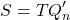K-map for input R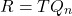From the above expressions, the input S is AND combination of T and Qn+1 and the input R is AND combination of T and Qn.

So, in the circuit diagram, we need two AND gates. And the circuit diagram of T flip flop from SR flip flop is as shown below figure.

### T Flip Flop Using D Flip Flop

T flip flop can also be derived from D flip flop. For this derivation, we need the truth table of T flip flop (table-1) and the excitation table of D flip flop.

From the reference of truth table of T flip flop and excitation table of D flip flop, we can make a conversion table as below.

Now, let’s make K-map for input D.

Here, we have a diagonal pair in K-map. Therefore, it will relate to the XOR sign. And the expiration of D in terms of T is as the below equation.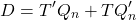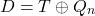So, the circuit diagram of T flip flop using D flip flop need XOR gate connected as the below circuit diagram.

Fig Circuit diagram of T flip flop using D flip flop

## T Flip Flop Timing Diagram

As we know, the T flip flop toggle the current state of the input. When T flip flop is activated (1) if the present state is high (1), the output will be low (1) and vice versa.

Assume the initial condition (at time T0) for a present state (Qn) is low and for the next state (Qn+1) is high.

At time T1, toggle (T) changes from low to high. So, the T flip flop is activated. In this condition, Qn and Qn+1 will change. Therefore, Qn will high and Qn+1 will low.

At time T2, Toggle (T) changes from high to low. And the output remains unchanged or hold.

At time T3, toggle (T) changes from low to high. In this condition, the device changes state. So, Qn goes high from low and Qn+1 goes low from high.

At time T4, toggle (T) changes from high to low. Output remains unchanged until time T5.

From the timing diagram, we can observe that the toggle (T) completed two cycles between time T1 to T5. During this same time of period, Qn and Qn+1 completed only one cycle. Hence, T flip flop is also used to divide the input by 2.

Want To Learn Faster? 🎓
Get electrical articles delivered to your inbox every week.
No credit card required—it’s 100% free.